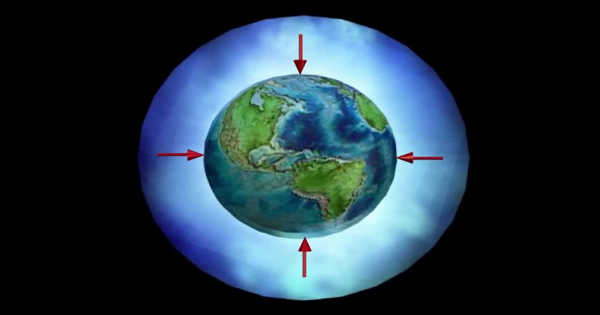Other

# Atmospheric Pressure – the Pressure within the Atmosphere of EarthAtmospheric pressure, also known as barometric pressure, is the pressure that exists within the Earth’s atmosphere. The standard atmosphere (abbreviated atm) is a pressure unit defined as 101,325 Pa (1,013.25 hPa; 1,013.25 mbar), which is equivalent to 760 mm Hg, 29.9212 inches Hg, or 14.696 psi. The atm unit is roughly equivalent to the average sea-level atmospheric pressure on Earth, i.e., the atmospheric pressure at sea level on Earth is approximately 1 atm.

The gravitational attraction of the planet on the atmospheric gases above the surface causes atmospheric pressure, which is a function of the planet’s mass, the radius of the surface, the amount and composition of the gases, and their vertical distribution in the atmosphere. It is influenced by planetary rotation as well as local effects such as wind velocity, density variations due to temperature, and composition changes.

Atmospheric pressure is defined as the force per unit area exerted against a surface by the weight of the air above that surface.

A barometer is commonly used to measure atmospheric pressure. As the weight of the atmosphere changes, a column of mercury in a glass tube rises or falls in a barometer. Meteorologists define atmospheric pressure based on how high the temperature rises. An atmosphere (atm) is a measurement unit equal to the average air pressure at sea level at 15 degrees Celsius (59 degrees Fahrenheit). One atmosphere has a pressure of 1,013 millibars or 760 millimeters (29.92 inches) of mercury.

In most cases, the hydrostatic pressure caused by the weight of air above the measurement point closely approximates atmospheric pressure. Because there is less overlying atmospheric mass as elevation increases, atmospheric pressure decreases as elevation increases.

Pressure is measured in SI units of pascals and represents force per unit area (1 pascal = 1 newton per square meter, 1 N/m2). A column of air with a cross-sectional area of 1 square centimeter (cm2), measured from the mean (average) sea level to the top of Earth’s atmosphere, has a mass of approximately 1.03 kilogram and exerts a force or “weight” of approximately 10.1 newtons, resulting in a pressure of 10.1 N/cm2 or 101 kN/m2. A column of air with a cross-sectional area of 1 in2 would have a weight of about 14.7 lbf, resulting in a pressure of 14.7 lbf/in2.

The force per unit area exerted by the weight of the atmosphere is defined as atmospheric pressure. Meteorologists use a barometer to determine this weight. Evangelista Torricelli, an Italian physicist, and mathematician demonstrated that he could weigh the atmosphere against a column of mercury in 1643. He actually measured pressure and converted it to weight. Torricelli’s invention was the world’s the first barometer. An open dish of mercury is placed in the open end of a glass tube. The mercury is forced up the tube by atmospheric pressure. At sea level, the mercury column will rise to a height of 29.92 inches (760 millimeters) on average.